UPSC  >  Exercise 14.4 NCERT Solutions - Statistics

# Exercise 14.4 NCERT Solutions - Statistics - NCERT Textbooks (Class 6 to Class 12) - UPSC

Question 1. The following number of goals were scored by a team in a series of 10 matches:
2, 3, 4, 5, 0, 1, 3, 3, 4, 3
Find the mean, median and mode of these scores.
Solution:

Mean = Average = Sum of all the observations/Total number of observations
= (2+3+4+5+0+1+3+3+4+3)/10

= 28/10

= 2.8

Median,
To find the median, we first arrange the data in ascending order.

0, 1, 2, 3, 3, 3, 3, 4, 4, 5

Here,

Number of observations (n) = 10

Since the number of observations are even, the median can be calculated as: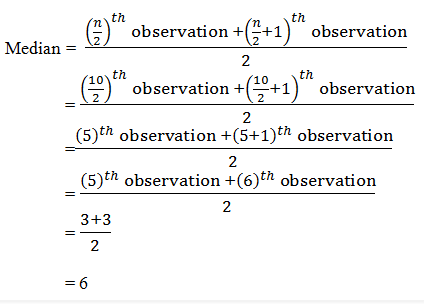= 3

Mode,

To find the mode, we first arrange the data in ascending order.

0, 1, 2, 3, 3, 3, 3, 4, 4, 5.

Here,

We find that 3 occurs most frequently (4 times)

∴ Mode = 3

Question 2. In a mathematics test given to 15 students, the following marks (out of 100) are recorded:
41, 39, 48, 52, 46, 62, 54, 40, 96, 52, 98, 40, 42, 52, 60
Find the mean, median and mode of this data.
Solution:

Mean=Average = Sum of all the observations/Total number of observations
= (41+39+48+52+46+62+54+40+96+52+98+40+42+52+60)/15
= 822/15
= 54.8
Median,
To find the median, we first arrange the data in ascending order.
39, 40, 40, 41, 42, 46, 48, 52, 52, 52, 54, 60, 62, 96, 98
Here,
Number of observations (n) = 15
Since the number of observations are odd, the median can be calculated as:
Median = [(n+1)/2]th observation
= [(15+1)/2]th observation
= (16/2)th observation
= 8th observation
= 52
Mode,
To find the mode, we first arrange the data in ascending order.
39, 40, 40, 41, 42, 46, 48, 52, 52, 52, 54, 60, 62, 96, 98
Here, We find that 52 occurs most frequently (3 times)

∴ Mode = 52

Question 3. The following observations have been arranged in ascending order. If the median of the data is 63, find the value of x.
29, 32, 48, 50, x, x+2, 72, 78, 84, 95

Solution:
Number of observations (n) = 10
Given that Median = 63
Since the number of observations are even, the median can be calculated as: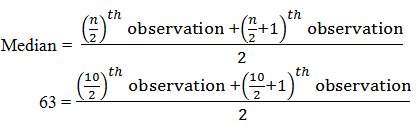63 = [5th observation+(5+1)th observation]/2
63 = [5th observation+6th observation]/2
63 = (x+x+2)/2
63 = (2x+2)/2
x = 63-1
x = 62

Question 4. Find the mode of 14, 25, 14, 28, 18, 17, 18, 14, 23, 22, 14, 18.
Solution:

Mode,
To find the mode, we first arrange the given data in ascending order.
14,14,14,14,17,18,18,18,22,23,25,28
Here,
We find that 14 occurs most frequently (4 times)
∴ Mode = 14

Question 5. Find the mean salary of 60 workers of factory from the following table: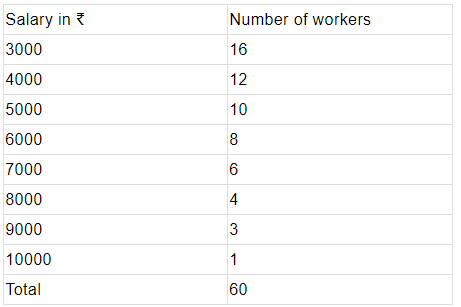Solution: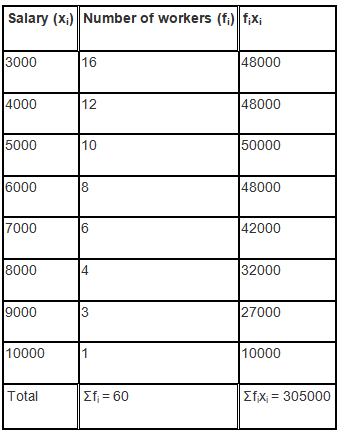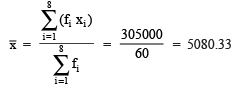Thus, the mean salary is ₹5083.33

Question 6. Give one example of a situation in which
(i) The mean is an appropriate measure of central tendency.
(ii) The mean is not an appropriate measure of central tendency but the median is an appropriate measure of central tendency.

Solution:

(i) Mean marks obtained in the examination

(ii) Runs scored by Mahendra Singh Dhoni in 7 matches are,
39, 51, 56, 102, 83, 48, 91
Here,
Mean = (39+51+56+102+83+48+91)/7
= 470/7
= 67.1.
Median,
Arranging in ascending order we get 39, 48 51, 56, 83, 91, 102
n = 7
Median = [(n+1)/2]th observation
= ( (7+1)/2)th observation
= (8/2)th observation
= 4th observation
= 56

The document Exercise 14.4 NCERT Solutions - Statistics | NCERT Textbooks (Class 6 to Class 12) - UPSC is a part of the UPSC Course NCERT Textbooks (Class 6 to Class 12).
All you need of UPSC at this link: UPSC

## NCERT Textbooks (Class 6 to Class 12)

1402 docs|679 tests

## FAQs on Exercise 14.4 NCERT Solutions - Statistics - NCERT Textbooks (Class 6 to Class 12) - UPSC

 1. What is the importance of statistics in research?Ans. Statistics plays a crucial role in research as it helps in collecting, organizing, analyzing, and interpreting data. It provides researchers with tools and techniques to make sense of the data they have collected, enabling them to draw meaningful conclusions and make informed decisions based on evidence.
 2. How is statistics used in business decision-making?Ans. Statistics is extensively used in business decision-making as it helps in forecasting future trends, evaluating risks, and making informed business strategies. It enables businesses to analyze market data, customer behavior, and financial information to identify opportunities, optimize resources, and improve overall performance.
 3. What are the different types of statistics?Ans. There are two main types of statistics: descriptive statistics and inferential statistics. Descriptive statistics involve summarizing and presenting data in a meaningful way, such as through measures of central tendency (mean, median, mode) and measures of dispersion (range, standard deviation). Inferential statistics, on the other hand, involve using data from a sample to make inferences or draw conclusions about a larger population.
 4. How does statistics help in understanding social phenomena?Ans. Statistics helps in understanding social phenomena by providing a quantitative framework to analyze and interpret social data. It allows researchers to study social trends, patterns, and relationships, and make evidence-based claims about various social phenomena. Whether it is studying demographic trends, social inequality, or public opinion, statistics provides a rigorous and systematic approach to understand and analyze social issues.
 5. What are the limitations of statistics?Ans. While statistics is a powerful tool, it has certain limitations. One limitation is that it can only provide information about the observed data and cannot establish causation. It can only identify correlations and associations between variables. Additionally, statistics relies on assumptions and simplifications, which may not always reflect the complex reality of a situation. It is also important to ensure the quality and reliability of data used in statistical analysis to avoid biased or misleading results.

## NCERT Textbooks (Class 6 to Class 12)

1402 docs|679 testsExplore Courses for UPSC exam### How to Prepare for UPSC

Read our guide to prepare for UPSC which is created by Toppers & the best Teachers
Signup to see your scores go up within 7 days! Learn & Practice with 1000+ FREE Notes, Videos & Tests.
10M+ students study on EduRev
Track your progress, build streaks, highlight & save important lessons and more!
Related Searches

,

,

,

,

,

,

,

,

,

,

,

,

,

,

,

,

,

,

,

,

,

;